# ML Wiki

## Taylor Series

The idea behind Taylor Series is that any well-behaving Function (e.g. Continuous Functions) can be represented as a sum of Polynomials

### Taylor and Maclaurin Series

A Taylor Series of $f(x)$ at $x=0$ is

• $f(x) = \sum\limits_{k=0}^\infty \cfrac{f^{(k)}(0)}{k!}\, x^k$
• where $f^{(k)}(0)$ is $k$th Derivative of $f$ evaluated at $x=0$
• this kind of Taylor Series about $x = 0$ is sometimes called Maclaurin Series

Taylor Series of $f(x)$ at $x = a$ is

• $f(x) = \sum\limits_{k=0}^\infty \cfrac{f^{(k)}(a)}{k!}\, (x - a)^k$
• this is the general form

### Expansion as an Operator

Taylor Expansion is the process of turning a function to a Taylor Series

• can think of it as an operator that takes a function and returns a Series
•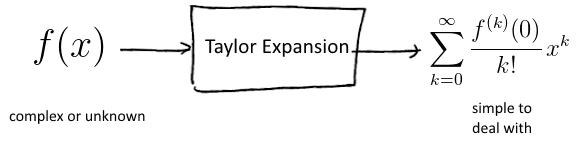### Examples

Famous expansions:

• Exponential Function: $e^x = \sum\limits_{k=0}^{\infty} \frac{1}{k!} x^k$ of Exponential Function
• Trigonometric Functions:
• $\cos x = \sum\limits_{k=0}^\infty (-1)^k \cfrac{x^{2k}}{(2k)!}$ of Cosine
• $\sin x = \sum\limits_{k=0}^\infty (-1)^k \cfrac{x^{2k + 1}}{(2k + 1)!}$ of Sine
• it allows us to deal with these functions as with long sums of polynomials

## Approximation

Taylor Series are used for approximations

Exponential

• as we add more terms, we are closer and closer to the function
•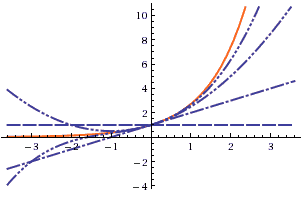(source: )
• order $n$ approximation is shown with $n$ dots

Trigonometric Functions:

•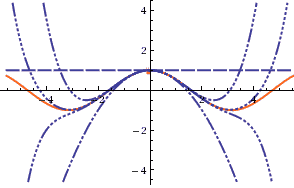(source: )
•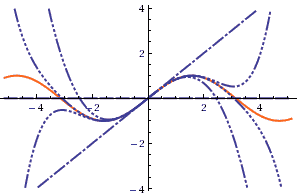(source: )
• by taking only polynomials of odd (even) powers, we get cosines or sines

Approximation near the expansion point 0:

• Note that these approximations work best near 0
• It's clear from the sin/cos graphs - the more away from 0, need more terms

## Computing Taylor Series

How to compute Taylor Series? There are several ways of doing it

### Derivatives

• This is the straightforward way:
• use the definitions and compute all the Derivatives
• if a function is complex, it may be hard

### Substitution

Example: $\cfrac{1}{x}\, \sin (x^2)$

• hard to take derivatives
• but we know the expansion of $\sin x$, so
• $\cfrac{1}{x}\, \sin (x^2) = \cfrac{1}{x}\, \left( (x^2) - \cfrac{1}{3!}\, (x^2)^3 + \cfrac{1}{5!}\, (x^2)^5 -\ ... \right) =\ ...$
• $... \ = x - \cfrac{1}{3!}\, x^5 + \cfrac{1}{5!}\, x^9 -\ ... = \sum\limits_{k=0}^\infty (-1)^k \cfrac{x^{4k + 1}}{(2k + 1)!}$

### Combination

$\cos^2 x = \cos x \cdot \cos x$

• expand each one separately
• $\cos x \cdot \cos x = \left(1 - \cfrac{1}{2!}\, x^2 + \cfrac{1}{4!}\, x^4 -\ ... \right)\, \left( 1 - \cfrac{1}{2!}\, x^2 + \cfrac{1}{4!}\, x^4 -\ ... \right) = \ ...$
• $... \ = 1\cdot 1 + 1\, \left(-\cfrac{1}{2!}\, x^2\right) + 1\, \left(\cfrac{1}{4!}\, x^4\right) + \ ... \ - \cfrac{1}{2}\, x^2 \cdot 1 - \cfrac{1}{2}\, x^2 \left(-\cfrac{1}{2!}\, x^2\right) - \cfrac{1}{2}\, x^2 \left(\cfrac{1}{4!}\, x^4\right) - \ ... \ = \ ...$
• $... \ = 1- x^2 +\cfrac{1}{3}\, x^4 - \cfrac{2}{45}\, x^6 + \ ...$
• since $\cos^2 x = 1 - \sin^2 x$, we also obtained the expansion of $\sin^2 x$!

## Higher Order Terms

Instead of writing "..." can just say "HOT" meaning "Higher order terms":

• $e^x = 1 + x + \cfrac{1}{2}\, x^2 + \cfrac{1}{3!}\, x^3 + \text{HOT}$
• we mean that we don't care what terms are there - it's fine to consider just leading terms for some purposes
• it can simplify our calculations

For example:

• $f(x) = 1 - 2x\, e^{\sin (x^2)}$
• How can we Taylor Expand it?
• $\sin (x^2) = x^2 - \cfrac{1}{3!}\, (x^2)^3 + \text{HOT} = x^2 - \cfrac{1}{3!}\, x^6 + \text{HOT}$
• $e^{\sin (x^2)} = 1 + \left( x^2 - \cfrac{1}{3!}\, x^6 + \text{HOT} \right) + \cfrac{1}{2!}\, \left( x^2 - \cfrac{1}{3!}\, x^6 + \text{HOT} \right)^2 + \cfrac{1}{3!}\, \left( x^2 + \text{HOT} \right)^3 + \text{HOT} = 1 + x^2 + \cfrac{1}{2}\, x^4 + \text{HOT}$
• so $f(x) = 1 - 2x\, e^{sin (x^2)} = 1 - 2x\, \left(1 + x^2 + \cfrac{1}{2}\, x^4 + \text{HOT} \right)$

To show what behavior HOTs have, we use Orders of Growth: the Big-O notation:

• $e^x\, \cfrac{1}{1-x} = \big(1 + x + O(x^2)\big) \cdot \big(1 + x + O(x^2)\big) = \ ...$
• $... \ = (1 + x)^2 + 2\, (1 + x)\, O(x) + \big( O(x^2)\big)^2 = 1 + 2x + O(x^2) + O(x^3) + O(x^4) = 1 + 2x + O(x^2)$

## Convergence

Series = adding an infinite number of terms

• it can be dangerous
• Problem: not all functions can be expressed as sum of Polynomial Functions, i.e. as $f(x) = \sum c_k x^k$

for example, natural Logarithm:

•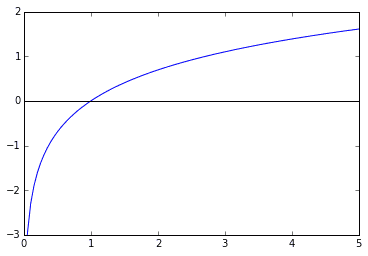• $\ln x$ is not even defined at $x=0$
• polynomials are too simple to capture all the complexity of $\ln x$

### Convergence Domain

Taylor Series has a convergence domain on which the series is well behaved

• for many functions, e.g. $e^x$, $\sin x$, $\cos x$, $\sinh x$, etc, the domain is $\mathbb R = (-\infty, \infty)$

Within the domain of convergence you can do with series whatever you want:

• rearrange terms
• differentiate/integrate
• combine

For example, $\ln (1 + x)$

• $\ln (1 + x) = \int \cfrac{1}{1 + x}\, dx$
• $\ln (1 + x) = \int \left(\sum\limits_{k=0}^\infty (-x)^k \right)\, dx = \ ...$
• can rearrange integral and sum:
• $... \ = \sum\limits_{k=0}^\infty \left( \int (-x)^k \, dx \right) = \ ...$
• $... \ = \sum\limits_{k=0}^\infty (-1)^k \cfrac{x^{k+1}}{k+ 1} + C = \ ...$
• $C = 0$ because $\ln 1 = 0$, so we have
• $\ln (1 + x) = \sum\limits_{k=1}^\infty (-1)^{k+1}\, \cfrac{x^k}{k} = x - \cfrac{1}{2}\, x^2 + \cfrac{1}{3}\, x^3 - \cfrac{1}{4}\, x^4 + \ ...$
• no factorials involved!

Convergence domain:

• we used Geometric Series here, so we must be in the domain of convergence of this series
• which is $|x| < 1$
• Taylor Series approximates well only on the domain of convergence
•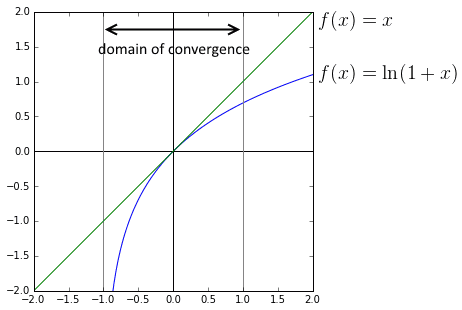• outside of the domain, we don't get better approximation when we add more terms!

Another example: $\arctan x$

• $\arctan x = \int \cfrac{1}{1 + x^2}\, dx$
• we also have a Geometric Series here, with $-x^2$, so
• $\arctan x = \int \cfrac{1}{1 + x^2}\, dx = \int \sum\limits_{k=0}^\infty (-x^2)^k \, dx = \ ...$
• $... \ = \int \sum\limits_{k=0}^\infty (-1)^k x^{2k} \, dx = \sum\limits_{k=0}^\infty \left( \int (-1)^k x^{2k} \, dx \right) = \ ...$
• $... \ = \sum\limits_{k=0}^\infty \cfrac{(-1)^k}{2k + 1}\, x^{2k + 1} + C$
• $C = 0$ because $\arctan 0 = 0$
• so $\arctan x = x - \cfrac{1}{3}\, x^3 + \cfrac{1}{5}\, x^5 - \cfrac{1}{7}\, x^7 + \ ...$
•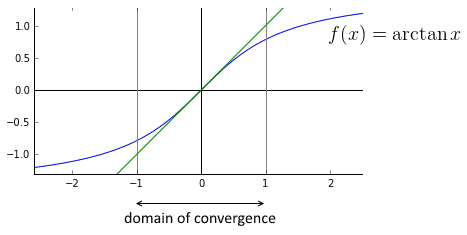• the domain of convergence is also $|x| < 1$

## Expansion Points

• Maclaurin Series give good approximation at points near 0.
• But what if we want to have good approximations at some other points?
• In many applications 0 is not the most interesting point

Taylor Series of $f(x)$ at $x = a$ is

• $f(x) = \sum\limits_{k=0}^\infty \cfrac{f^{(k)}(a)}{k!}\, (x - a)^k = f(a) + \cfrac{df}{dx}|_{a} (x - a) + \cfrac{1}{2!}\, \cfrac{d^2f}{dx^2}|_{a} (x - a)^2 + \cfrac{1}{3!}\, \cfrac{d^3f}{dx^3}|_{a} (x - a)^3 + \ ...$
• this is polynomial in $(x - a)$ instead of just $x$

### Approximation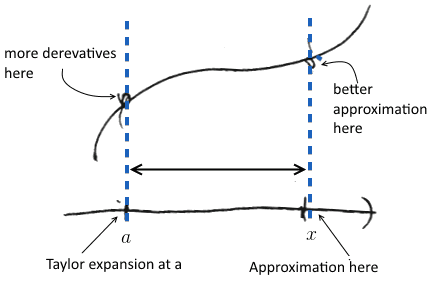• The bigger $|a - x|$ is, the more high-order terms we need to have good approximation
• Taylor Series converge only within the domain of convergence - so need to stay within the domain

Example:

• estimate $\sqrt{10}$
• $\sqrt{x}$ expanded at $x = a$ is $\sqrt{x} = \sqrt{a} + \cfrac{1}{2\, \sqrt{a}}\, (x - a) - \cfrac{1}{8\, \sqrt{a^3}}\, (x - a)^2 + \text{HOT}$
• let's approximate it at $a = 1$:
• $\sqrt{x} = 1 + \cfrac{1}{2}\, (x - 1) - \cfrac{1}{8}\, (x - 1)^2 + \text{HOT}$
• for $x = 10$, we get $\approx -4.122$
• bad approximation! $a = 1$ is far from $x = 10$, so need more derivatives to approximate better
• let's consider $a = 9$ ($\sqrt{9} = 3$)
• $\sqrt{x} = 3 + \cfrac{1}{6}\, (x - 9) - \cfrac{1}{216}\, (x - 9)^2 \approx -4.122$
• for $x = 10$ we get $3 + 1/6 - 1/216 \approx 3.1620$ (real is $3.1623$)
• quite close!
• so it's important to select $a$ which is close to the value $x$ where you want to approximate $f(x)$

### Taylor Expansion via Decomposition

Be careful with expansion points when doing Taylor Expansion via decomposition Expand about the correct value!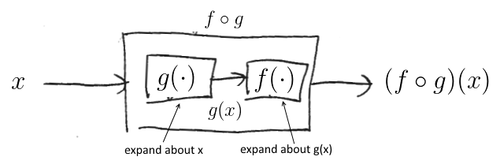For example, compute expansion for $e^{\cos x}$ about $x = 0$

• we have: $0 \to \cos (\cdot) \to e^{(\cdot)} \to$
• first, we expand about 0
• but for $e$, we don't expand about 0 - we expand about $\cos 0 = 1$!
• $\cos x = 1 - \cfrac{1}{2!}\, x^2 + \cfrac{1}{4!}\, x^4 - \ ...$
• $e^u = e + e\, (u - 1) + \cfrac{1}{2!}\, e\, (u - 1)^2 + \ ...$